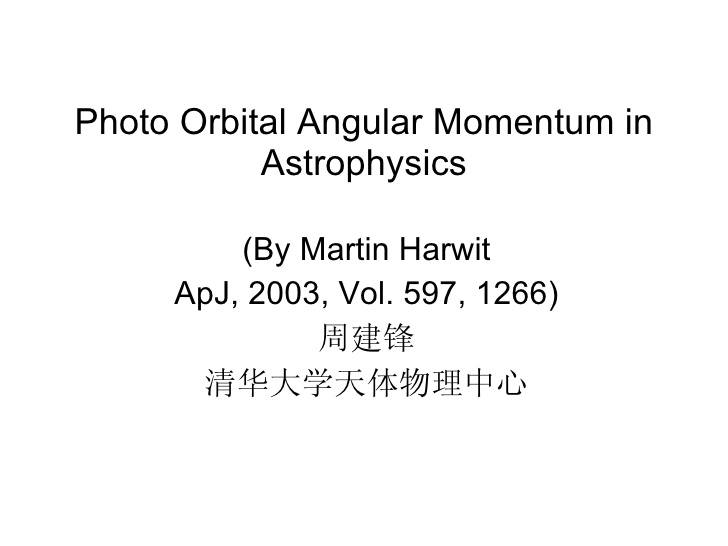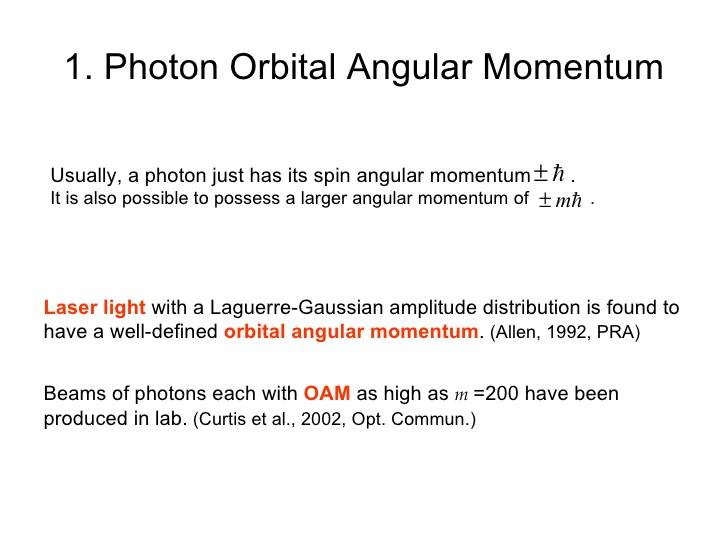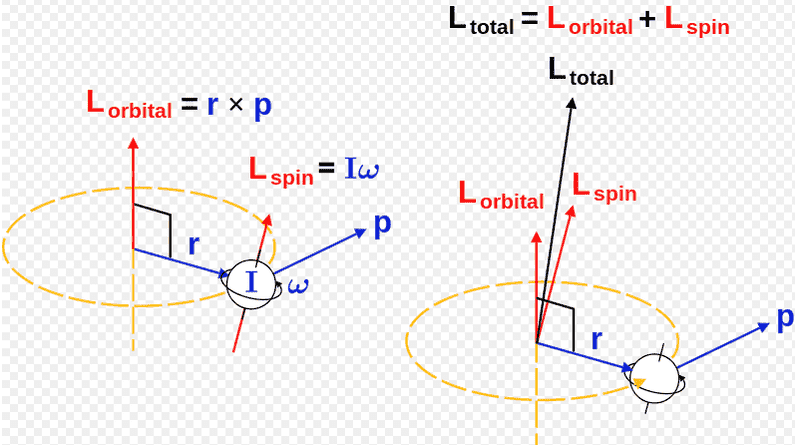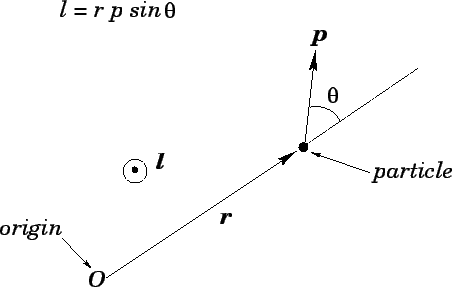# Orbital angular momentum of light

• I
Gold Member
A spiral phase plate can change the orbital angular momentum of a beam of light. Should I think of the beam of light carrying the orbital angular momentum or the photons that make up the beam light?

If the orbital angular momentum is carried by the individual photons what is being orbited, the axis of propagation? Seems to me like it would have to have some kind of "cork screw" motion? Is there a simple way to see this while being true to quantum optics?

Thanks!

## Answers and Replies

A spiral phase plate can change the orbital angular momentum of a beam of light. Should I think of the beam of light carrying the orbital angular momentum or the photons that make up the beam light?

If the orbital angular momentum is carried by the individual photons what is being orbited, the axis of propagation? Seems to me like it would have to have some kind of "cork screw" motion? Is there a simple way to see this while being true to quantum optics?

Thanks!
This Wiki article seems reasonably accurate for the classical definitions ( in case you missed it ).

Gold Member
This Wiki article seems reasonably accurate for the classical definitions ( in case you missed it ).

I read it again and did not see my questions addressed. I suspect that it must be that it is the individual photons which carry the orbital angular momentum, again orbiting what? I guess there must be some non-trivial quantum optics operator which will produce a single photon with one unit of orbital angular momentum?

Edit, maybe Wiki answers my questions but it is not obvious to me, maybe obvious to Mr.(?) Mentz however.

Thanks!

Last edited:
I read it again and did not see my questions addressed. I suspect that it must be that it is the individual photons which carry the orbital angular momentum, again orbiting what? I guess there must be some non-trivial quantum optics operator which will produce a single photon with one unit of orbital angular momentum?

Edit, maybe Wiki answers my questions but it is not obvious to me, maybe obvious to Mr.(?) Mentz however.

Thanks!
No, the Wiki article is mostly classical. Quantum spin does not have the simple interpretation that classical spin has. 'Orbiting around what' is not a meaningful question.
In Gerry & Knight Introductory Quantum Optics an operator is defined ##j_3=(a_0^{\dagger} a_0 - a_1^{\dagger} a_1)/2## which has the same commutation relations as spin ( ##\left[ j_\alpha, j_\beta \right] = i \epsilon_{\alpha\beta\gamma} j_\gamma##) and that is all I can tell you about photons and angular momentum.

If I may call on @Simon Phoenix I'm sure he understands all this.

Last edited:
•Spinnor
vanhees71
Science Advisor
Gold Member
This Wiki article seems reasonably accurate for the classical definitions ( in case you missed it ).
Hm, I'm a bit puzzled by this Wiki article. It's not obviously wrong, but may be misleading. If you read it carefully (and supposed it isn't wrong, which I haven't checked carefully myself yet), it should come out that what's well defined is the total angular momentum, and that's the expression given in the beginning (rewritten in Heaviside Lorentz units)
$$\vec{J}_{\text{em}}=\frac{1}{c}\int_{\mathbb{R}^3} \vec{r} \times \vec{E} \times \vec{B}.$$
The splittings into "orbital" and "spin" angular momentum are at least questionable since, as the expressions in the Wiki article show, are gauge dependent and thus cannot be interpreted in a physically well-defined way.

•Mentz114
vanhees71
Science Advisor
Gold Member
No, the Wiki article is mostly classical. Quantum spin does not have the simple interpretation that classical spin has. 'Orbiting around what' is not a meaningful question.
In Gerry & Knight Introductory Quantum Optics an operator is defined ##j_3=(a_0^{\dagger} a_0 - a_1^{\dagger} a_1)/2## which has the same commutation relations as spin ( ##\left[ j_\alpha, j_\beta \right] = i \epsilon_{\alpha\beta\gamma} j_\gamma##) and that is all I can tell you about photons and angular momentum.

If I may call on @Simon Phoenix I'm sure he understands all this.
In Landau Lifshitz vol. IV you find a proper description of the spherical-wave basis for the single-photon and thus also for the Fock space of free photons. It's analgous to the well-known multipole expansion of the electromagnetic field in terms of vector spherical harmonics.

•Mentz114
Gold Member
So if you can believe,Then is looks like it is the photon that carries the orbital angular momentum,From, https://www.google.com/imgres?imgurl=https://image.slidesharecdn.com/poam-101219235506-phpapp02/95/poam-6-728.jpg?cb=1292803887&imgrefurl=https://www.slideshare.net/ZenBossanova/poam-6248261&docid=YnBJ8J9VqXMcpM&tbnid=9UrmjQMfSRhYwM:&vet=10ahUKEwjErfCT49TTAhWL0YMKHQikCNwQMwhvKEAwQA..i&w=728&h=546&hl=en&safe=off&bih=669&biw=1396&q=orbital angular momentum light&ved=0ahUKEwjErfCT49TTAhWL0YMKHQikCNwQMwhvKEAwQA&iact=mrc&uact=8

As for orbiting what I will leave that as a bad question.

See also Physics Today May 2004, Light's Orbital Angular Momentum.

Thanks!

Last edited:
vanhees71
Science Advisor
Gold Member
Again: A photon carries total angular momentum. It's not possible to split it into a spin and orbital angular momentum in a well-defined gauge-invariant way.

That's what you (should) learn in Theoretical Physics 3 under the name "multipole expansion of the radiation field". It starts with the dipole (the "monopole" component is necessarily static and thus no radiation component).

Gold Member
Again: A photon carries total angular momentum. It's not possible to split it into a spin and orbital angular momentum in a well-defined gauge-invariant way.

Can we chose a reference frame such that the total angular momentum of the photon reduces to just the spin of the photon? Spin angular momentum seems intrinsic to the photon and orbital angular momentum seems to depend on our choice of coordinates?

Thanks.

vanhees71
Science Advisor
Gold Member
Can we chose a reference frame such that the total angular momentum of the photon reduces to just the spin of the photon? Spin angular momentum seems intrinsic to the photon and orbital angular momentum seems to depend on our choice of coordinates?

Thanks.
A photon has not a spin as a massive particle. "It's a spin 1 particle" means that it is described by a vector field, but since it is massless it has only two polarization degrees of freedom (instead of three for a massive vector particle, which has a true spin, namely the angular momentum in its rest frame, and the corresponding spin-#z# component in this frame can take the three values +1, 0, and -1). Since a photon is massless, there is no rest frame, where you can define the spin.

The only intrinsic spin-like quantity which is independent of the reference frame is helicity, and a photon has helicity +1 and -1. These are the left- and right-circular polarization components. Note that the helicity is the projection of the total (!) angular momentum on the direction of the momentum of the photon. So it's a well-defined gauge independent Lorentz-invariant quantity.

For a massive particle helicity is of course not Lorentz invariant, because you can always flip its value by boosting in another reference frame! The reason that it is Lorentz invariant for a massless particle is that you cannot "overtake" it, because there's no reference frame which is faster than the speed of light relative to any proper frame (that's the fundamental principle of relativity that a massless particle always goes with the speed of light from the point of view of any observer).

Simon Phoenix
Science Advisor
Gold Member
•Mentz114, Spinnor and BvU
Gold Member
Thank you all. I need to go back and study more and maybe my questions will go away. I am looking at this through a classical lens and I guess there are problems with that (but ultimately want to understand this in terms of quantum physics). Consider the following image showing the pictorial sum of intrinsic and orbital angular momentum,It seems by changing our origin we can change the amount of orbital angular momentum but not the amount of intrinsic angular momentum? So with that classical picture in mind I wonder if orbital angular momentum in our photon is just a matter of which reference frame you chose? Will read more and try and come up with answers and better questions.

Better picture showing the dependence of angular momentum on choice of origin,Thanks!

Last edited: